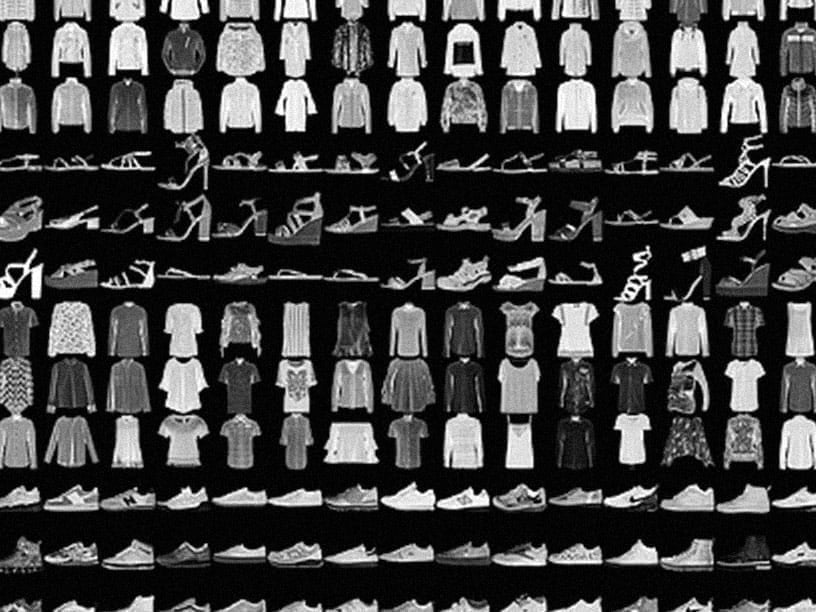TensorFlow is an end-to-end open source platform for machine learning.

TensorFlow makes it easy for beginners and experts to create machine learning models. See the sections below to get started.For beginners

The best place to start is with the user-friendly Sequential API. You can create models by plugging together building blocks. Run the “Hello World” example below, then visit the tutorials to learn more.

```import tensorflow as tf
mnist = tf.keras.datasets.mnist

x_train, x_test = x_train / 255.0, x_test / 255.0

model = tf.keras.models.Sequential([
tf.keras.layers.Flatten(input_shape=(28, 28)),
tf.keras.layers.Dense(128, activation='relu'),
tf.keras.layers.Dropout(0.2),
tf.keras.layers.Dense(10, activation='softmax')
])

loss='sparse_categorical_crossentropy',
metrics=['accuracy'])

model.fit(x_train, y_train, epochs=5)
model.evaluate(x_test, y_test)
```
For experts

The Subclassing API provides a define-by-run interface for advanced research. Create a class for your model, then write the forward pass imperatively. Easily author custom layers, activations, and training loops. Run the “Hello World” example below, then visit the tutorials to learn more.

```class MyModel(tf.keras.Model):
def __init__(self):
super(MyModel, self).__init__()
self.conv1 = Conv2D(32, 3, activation='relu')
self.flatten = Flatten()
self.d1 = Dense(128, activation='relu')
self.d2 = Dense(10, activation='softmax')

def call(self, x):
x = self.conv1(x)
x = self.flatten(x)
x = self.d1(x)
return self.d2(x)
model = MyModel()

logits = model(images)
loss_value = loss(logits, labels)
```
Solutions to common problemsFor beginners

Train a neural network to classify images of clothing, like sneakers and shirts, in this fast-paced overview of a complete TensorFlow program.For experts

Train a generative adversarial network to generate images of handwritten digits, using the Keras Subclassing API.For experts
Neural machine translation with attention

Train a sequence-to-sequence model for Spanish to English translation using the Keras Subclassing API.

News & announcementsTest Drive TensorFlow 2.0 AlphaFeb 27, 2019

TensorFlow 2.0 will include many API changes, such as reordering arguments, renaming symbols, and changing default values for parameters. To streamline the changes, the TensorFlow engineering team has created a tf_upgrade_v2 utility that will help transition legacy...Feb 26, 2019
Introducing TensorFlow Datasets

We’re pleased to introduce TensorFlow Datasets, which exposes public research datasets as tf.data.Datasets and as NumPy arrays.Dec 11, 2018
Try TensorFlow 2.0 in the Cloud

Google Cloud Deep Learning VM Images come pre-installed with everything you need to quickly get your TensorFlow 2.0 project started.

Community participation

See more ways to participate in the TensorFlow community.

TensorFlow on GitHub